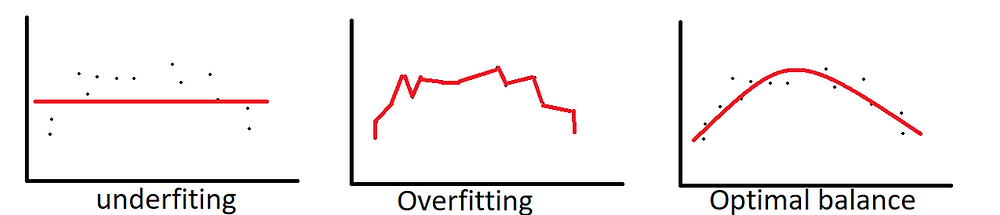Search
•Mani Abedini

# Overview of Bias–Variance Errors

Updated: Jul 19, 2020

Bias and Variance and their trade-off is an interesting Machine Learning topic. Understanding the concept will help building accurate model, either by choosing the right machine learning method, or how to tuning the learning parameter to avoid overfitting and underfitting problems.

Typically, the prediction error of machine learning models is composed of three errors:

Bias Error is the average difference between the model predictions and target (true) values. The bias error fundamentally caused by over simplifying the model and underestimating the complexity of the decision boundary to make it easier to learn. It usually leads to underfitting of the model and high learning error during training and test phase.

Variance Error is the variability of the model predictions for a given data point. Variance error caused by using unnecessary complex machine learning algorithm. The algorithm pays too much attention to training data, instead of focusing on generalization. As a result, it learns noise in training data. Variance leads to overfitting and high sensitivity to training steps, in other words if we go through different training scenarios and/or use different variation of the training data, we observe variation in predictions for the same given data point.

Irreducible Error is inevitable error; regardless of the learning method, hyper parameter tuning and the best training process. Since we cannot avoid irreducible error, lets focus on Bias and Variance and their trade -off.Bias vs Variance:

Generally, linear machine learning models have high bias due to over simplifying the model and have low learning capacity for complex problems.

On the other hand, non-linear machine learning model have tendency to high variance because of high learning capacity and sensitivity to the training data.

For example, Linear regression, and Logistic Regression has High Bias and Low Variance.

Decision tree, SVM and K Nearest Neighbour (K-NN) has Low Bias and High Variance.

There is a trade off between Bias and Variance, and there is a sweet optimal point in between. For example, as we discussed, k-NN has low bias but high variance, but by increasing the parameter k, more neighbours contribute to the final decision which will increase the bias of the model. Similarly, SVM has also low bias and high variance, increasing the parameter C, increases the Bias.

Decision tree has low Bias but high variance, because decision tree is very sensitive to when split the node based on training data. Random forest by introducing multiple variations of training data (bootstrapping) and random feature selection (bagging), reduce the sensitivity to training data, hence decrease Variance, while maintaining low Bias.